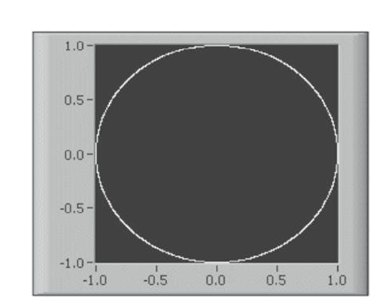# Waveform Data Type And XY Graphs In LABVIEW

Rating: 5

7090

## Waveform Data Type

The WAVEFORM data type carries the data, start time, and delta t of a waveform. You can create a waveform using the Build Waveform function. Many of the VIs and functions you use to acquire or analyze waveforms accept and return waveform data by default. When you wire waveform data to a waveform GRAPH or chart, the graph or chart automatically plots a waveform based on the data, start time, and delta x of the waveform. When you wire an array of waveform data to a waveform graph or CHART, the graph or chart automatically plots all waveforms.

## XY Graphs

The XY graph is a general-purpose, Cartesian graphing object that plots multivalued functions, such as circular shapes or waveforms with a varying time base. It displays any set of points, evenly sampled or not. You can also display Nyquist planes, Nichols planes, S planes, and Z planes on the XY graph. Lines and labels on these planes are the same color as the Cartesian lines, and you cannot modify the plane label font. The below Figure shows an example of an XY graph. The XY graph can display plots containing any number of points. It also accepts several data types, which minimizes the extent to which you must manipulate data before you display it.

If you would like to Enrich your career with an Android certified professional, then visit Mindmajix - A Global online training platform: “LabVIEW training” Course.This course will help you to achieve excellence in this domain.## Displaying A Single Plot On XY Graphs

The XY graph accepts three data types for single-plot XY graphs. The XY graph accepts a CLUSTER that contains an x array and a y array. The XY graph also accepts an array of points where a point is a cluster that contains an x value and a y value. It also accepts an array of complex data in which the real part is plotted on the x-axis and the imaginary part is plotted on the y-axis.## Displaying Multiple Plots On XY GraphS

The XY graph accepts three data types for displaying multiple plots. It accepts an ARRAY of plots where a plot is a cluster that contains an x array and a y array. The XY graph also accepts an array of clusters of plots where a plot is an array of points. A point is a cluster that contains an x value and a y value. The XY graph also accepts an array of clusters of plots where a plot is an array of complex data, in which the real part is plotted on the x-axis and the imaginary part is plotted on the y-axis.

Check Out LabVIEW TutorialsCourse Schedule
NameDates
LabVIEW TrainingFeb 11 to Feb 26
LabVIEW TrainingFeb 14 to Mar 01
LabVIEW TrainingFeb 18 to Mar 05
LabVIEW TrainingFeb 21 to Mar 08
Last updated: 08 February 2023